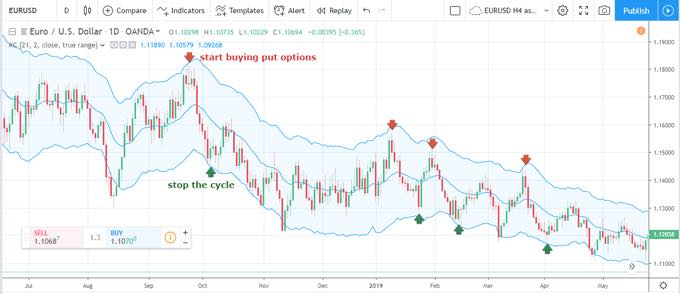The vast majority of effort spent by a technical analyst to assess current market conditions is related to finding an asset price that is close to a balanced value. In simple words, the technical analysis aims to calculate an average rate in a given period. With that in mind, traders compare the current price action to the average mean, making conclusions about the direction of a trend, its strength and momentum, measuring volatility and the speed of price action, and predicting the future market sentiment, using the Keltner Channel (mt4).

However, given the frequent fluctuations of financial assets and often whipsaws reflecting false movements, it becomes a tough challenge to get a precise figure of an average rate. This is why technical analysts always focus on ranges or bands, which are crucial for the overall equation at the end of the day. Effectively, that is why technical indicators became so popular in binary options trading. This article aims to provide an in-depth guide for one of the most effective envelope indicators, the Keltner Channel.

Keltner Channel explained
The strategy is based on a multi-purpose indicator, which shows the market volatility, trend’s direction, and strength, oversold and overbought conditions, as well as the average balanced price level. It is very similar to the widely used Bollinger Bands indicator, however, Chester Keltner – the developer of the indicator – represented a different way of measuring average ranges in the 1960s. His initial formula was based on Simple Moving Averages, but in the 1980s, a new method was offered – Exponential Moving Average and Average True Range.

THE FORMULA BEHIND THE AVERAGE
Before getting into the details of how the calculation is made, it’s worth explaining several crucial methods used in the formula, as well as their advantages in terms of practical use in binary options trading. Exponential Moving Average (EMA), in contrast to simple Moving Average (MA), has an additional constant of smoothing values. In other words, EMA is much more sensitive to price changes than SMA, and it has a less lagging distance. Thus, using EMAs in any indicator’s formula allows technical analysts to make more accurate predictions of resistance & support levels, breakthrough ranges, and reach a higher accuracy of the indicator as a result.

Average True Range (ATR) has even more layers of smoothing out unnecessary market noise. The formula picks up a maximum value between the difference in a high and low rate, absolute means of high rate less the previous close, and absolute mean of low price less previous close. After that, ATR is calculated by dividing 1 by the number of periods and multiplied by the sum of all previous readings for the chosen period. The main advantage of ATR compared to SMA is that it excludes false whipsaws and too large spikes of the price to calculate ranges where the rate was present more often. As a result, ATR points to a more probable price range in the future.

For more this success strategy, visit: FinmaxThis site uses Akismet to reduce spam. Learn how your comment data is processed.